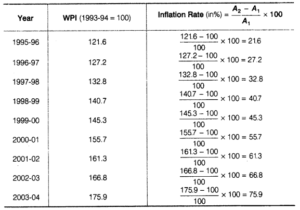# Chapter 8 – Index Numbers Questions and Answers: NCERT Solutions for Class 11 Economics(Statistics for Economics)

Class 11 Economics(Statistics for Economics) NCERT book solutions for Chapter 8 - Index Numbers Questions and Answers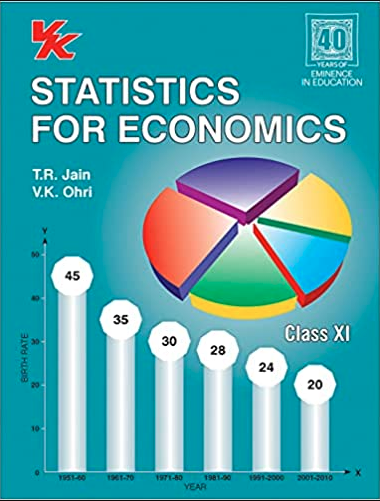## Question 14. The monthly per capita expenditure incurred by workers for an industrial centre during 1980 and 2005 on the following items are given below. The weights of these items are 75, 10, 5, 6 and 4 respectively. Prepare a weghted index number for cost of living for 2005 with 1980 as the base.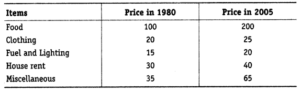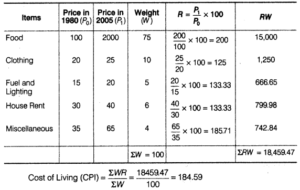### Answer: Index of Industrial Production Base 1993-94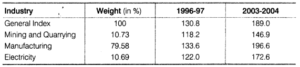## Question 18. The consumer price index for June, 2005 was 125. The food index was 120 and that of other items What is the percentage of the total weight given to food?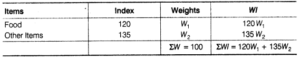### Let the total weight = 100 W1denotes weight of food W2denotes weight of other items So,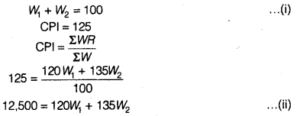### Multiplying both sides of Eq. (i) by 135 and subtracting Eq. (ii) from (i) we get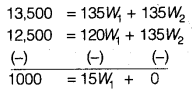## Question 19. An enquiry into the budgets of the middle class families in a certain city gave the following information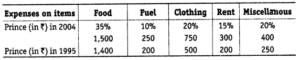## What is the cost of living index of 2004 as compared with 1995?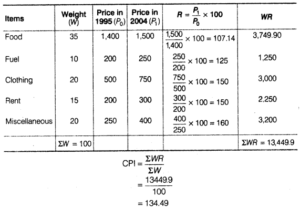## Question 21. Given the following data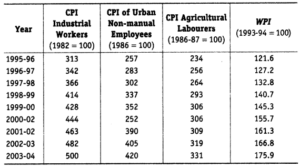## Source Economic Survey, Government of India 2004-2005 (i) Calculate the inflation rates using different index numbers. (ii) Comment on the relative values of the index numbers. (iii) Are they comparable?

### Answer: (i) (a) Inflation using CPI of Industrial Workers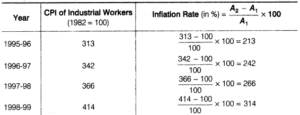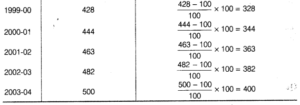### (b) Inflation using CPI of Non-maunal Employees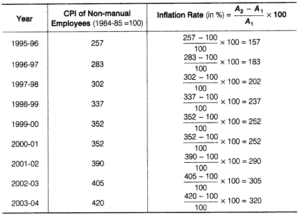### (c) Inflation using CPI of Agricultural Labourers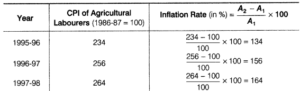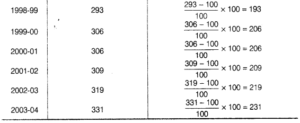### (d) Inflation using WPI